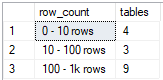## Number of tables by the number of rows in Azure SQL Database

Article for:

If you want to get an overview of how many rows the tables have in your database, one way is to count them by row intervals. This query returns the number of tables by the number of rows grouped in predefined intervals.

## Query

``````select
row_count,
count(*) tables
from
(select
[table],
case when rows > 1000000000 then '1b rows and more'
when rows > 1000000 then '1m - 1b rows'
when rows > 1000 then '1k - 1m rows'
when rows > 100 then '100 - 1k rows'
when rows > 10 then '10 - 100 rows'
else  '0 - 10 rows' end as row_count,
rows as sort
from
(
select schema_name(tab.schema_id) + '.' + tab.name as [table],
sum(part.rows) as [rows]
from sys.tables as tab
inner join sys.partitions as part
on tab.object_id = part.object_id
where part.index_id IN (1, 0) -- 0 - table without PK, 1 table with PK
group by schema_name(tab.schema_id) + '.' + tab.name
) as q
) as a
group by row_count
order by max(sort)
``````

## Columns

• row_count - predefined row count intervals:
• 0 - 10 rows
• 10 - 100 rows
• 100 - 1k rows
• 1k - 1m rows
• 1m - 1b rows
• 1b rows and more
• tables - number of tables whose row count falls in that interval

## Rows

• One row: represents one interval
• Scope of rows: all row count intervals that appear in the database
• Ordered by: from smallest tables to the largest

## Sample results

Here is a number of tables by row count in the AdventureWorksLT database grouped in predefined intervals.